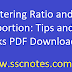Ratio and proportion are essential mathematical concepts that are used in many fields, including finance, engineering, science, and medicine. A ratio is a comparison of two quantities expressed as a fraction, while proportion is an equality of two ratios.

To solve problems involving ratio and proportion, it's essential to understand the relationship between the terms and use them to create an equation. Here are some tips that might help:

1. Understand the language of ratio and proportion. Words like "is to" and "per" indicate ratios, while words like "equals" and "proportional" indicate proportion.

2. Reduce ratios to their simplest form. It can make calculations easier and help you compare different ratios.

3. Cross-multiply when solving proportions. That means multiplying the numerator of one ratio by the denominator of the other ratio, and vice versa.

4. Be careful when working with percentages. They are just another way of expressing ratios, with a denominator of 100.

5. Practice, practice, practice! The more problems you solve, the better you'll become at ratio and proportion.

Mastering Ratio and Proportion: Tips and Tricks PDF DownloadReviewed by SSC NOTES on October 09, 2023 Rating: 5